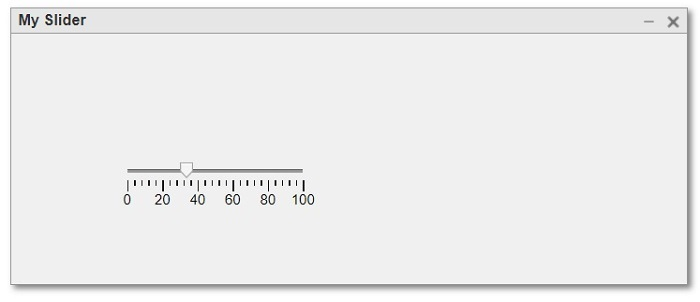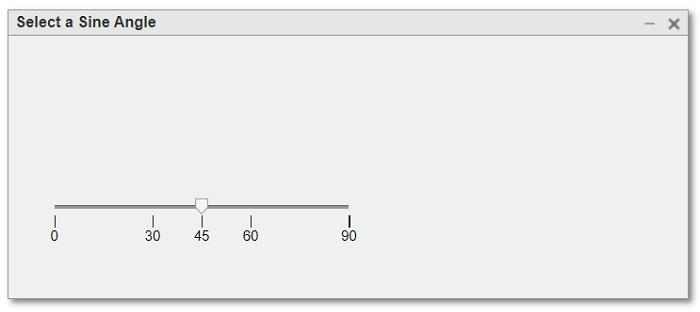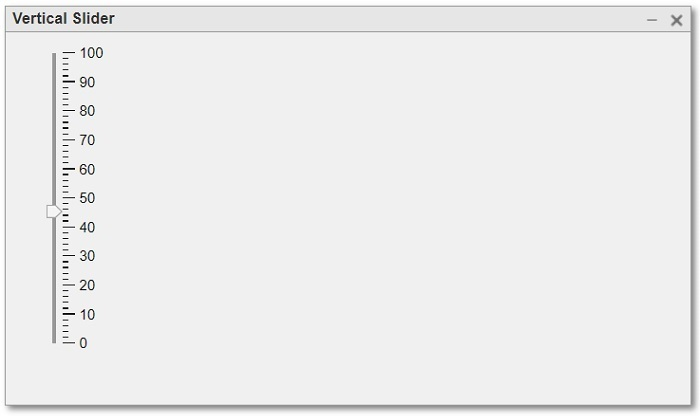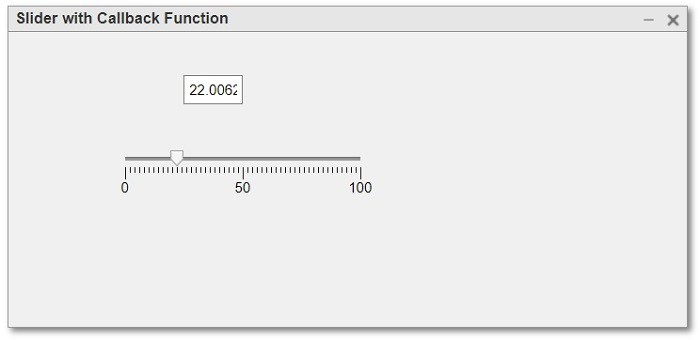# Create a Slider Component in MATLAB

MATLAB is a high-level programming language that allows us to create GUI applications without need of proper programming knowledge. In a MATLAB application, we can create various kinds of GUI (Graphical User Interface) components such as buttons, slider, text and number fields, hyperlinks, and many more.

This tutorial is meant for explaining the process of creating a slider component in a MATLAB application. A slider component in MATLAB application is a GUI component that allows users to chose a specific value from a range just by dragging a slider/wiper control along the range track. In MATLAB, we can create a slider component by using a built-in function 'uislider'. The slider is an interactive and user-friendly tool to input numeric values, control parameters, etc.

### Properties of Slider Compoennt in MATLAB

In MATLAB, a slider component has the following key properties −

• Limits − By using the limits property, we can specify the minimum and maximum values i.e., range of the slider.

• Value − This property of the slider component is used to set a default value of the slider. However, the user can change the value selected by dragging the slider control.

• Ticks and Labels − Ticks and labels property is used to indicate the divisions on the slider track which helps users to select a value easily.

• Orientation − This property allows to create the slider component either in horizontal direction or vertical direction.

• Callbacks − This property allows to assign an action to the slider component which responds to the user inputs.

• Appearance − This property allows to change the font, font color, width, height, etc. of the slider component.

After getting a brief overview of slider component, let us now see how to implement it in a MATLAB application.

## Slider Component in MATLAB

### Syntax

As mentioned above, MATLAB has a built-in function 'uislider' which is used to implement a slider component in a MATLAB application. This function has the following syntax −

slider_name = uislider(parameters);


Here, the parameters of the slider function are optional.

The following MATLAB programs demonstrate implementation of different kinds of sliders.

### (1). Slider Component with Default Properties

To create a slider component with default properties in MATLAB, we can use the following syntax of the 'uislider' function.

s = uislider;


Refer the following MATLAB program to understand the implementation of this syntax.

#### Matlab Example (1)

% MATLAB code to create a simple slider without properties
% Create a UI figure window
fig = uifigure('Name', 'My Slider');

% Create and display the slider
s = uislider(fig);


#### Output#### Explanation

This MATLAB program creates a UI figure window containing a slider with default properties.

### (2). Slider Component with Specified Properties

The following syntax of the 'uislider' function can be used to create a UI slider component with customized properties.

>s = uislider(---, 'PropertyName', 'PropertyValue', …);


Here, the parameter 'PropertyName' will be a property of the slider component like Position, Limits, Orientation, etc. and the parameter 'PropertyValue' will be the value of the specified property.

Consider the following MATLAB program to understand the implementation of this syntax.

#### Matlab Example (2)

% MATLAB program to create a slider component with specified properties
% Create a UI figure as a parent container
fig = uifigure('Name', 'Select a Sine Angle');

% Create a slider component
s = uislider(fig, 'Position', [40, 80, 250, 3], 'Limits', [0, 90], 'Value', 45, 'MajorTicks', [0, 30, 45, 60, 90], 'MinorTicks', []);


#### Output#### Explanation

This MATLAB code will display a UI figure window with a slider component. The range of this slider component will be from 0 to 90 and its slider control's default value will be 45.

### (3). Create a Vertical Slider Component

To create a vertical slider component, we have to specify its orientation property as vertical. The following program demonstrates how to create a vertical slider component.

#### Matlab Example (3)

% MATLAB program to create a vertical slider component
% Create a UI figure as a parent container
fig = uifigure('Name', 'Vertical Slider');

% Create a slider component
s = uislider(fig, 'Position', [40, 80, 250, 3], 'Limits', [0, 100], 'Value', 45, 'Orientation', 'Vertical');


#### Output#### Explanation

This MATLAB program creates a vertically oriented slider component in a UI figure window.

### (4). Slider Component with Callback Function

The following MATLAB program demonstrates the implementation of a slider component in MATLAB with an action assigned to it.

In this example program, we will implement an action that when the slider control is moved on the slider track, the value pointed by the control will reflect in a number field.

#### Matlab Example (4)

% MATLAB program to create slider component with callback function
% Create a figure as a parent container
fig = uifigure('Name', 'Slider with Callback Function');

% Create a number field
Number_Field = uieditfield(fig, 'Position', [150, 200, 50, 25]);

% Create a slider component
s = uislider(fig, 'Position', [100, 150, 200, 3], 'Limits', [0, 100], 'Value', 50, 'MajorTicks', [0, 50, 100]);

% Callback function to update number field
s.ValueChangedFcn = @(source, event) UpdateNumField(source, Number_Field);

% Callback function to update number field
function UpdateNumField(slider, Number_Field)
Number_Field.Value = num2str(slider.Value);
end


#### Output#### Explanation

This MATLAB program creates a slider component with a number field to show the selected value on the slider track.

The most important thing to consider in this program that if you are running this code in MATLAB editor, then make sure to save the callback function (UpdateNumField) in a separate file with .m extension, and if you are running the code in the Command Window, you can specify it within the same script.

## Conclusion

This is all about creating a slider component in MATLAB. In this tutorial, we demonstrated how to create a slider component in MATLAB with the help of example programs.

Updated on: 06-Sep-2023

38 Views